Import relevant packages

#collapse-hide

import matplotlib.pyplot as plt
import numpy as np
import pandas as pd
from functools import reduce
import re
import probscale
import seaborn as sns
sns.set(style="ticks", font_scale=1.5)
from pandas.plotting import register_matplotlib_converters
register_matplotlib_converters()
import urllib.request

Go to NOAA's National Centers for Environmental Information (NCEI)
Climate Data Online: Dataset Discovery

Find station codes in this map. On the left, click on the little wrench next to "Global Summary of the Month", then click on "identify" on the panel that just opened, and click on a station (purple circle). You will see the station's name, it's ID, and the period of record. For example, for Ben-Gurion's Airport in Israel:
BEN GURION, IS
STATION ID: ISM00040180
Period of Record: 1951-01-01 to 2020-03-01

You can download daily or monthly data for each station. Use the function below to download this data to your computer. station_name can be whatever you want, station_code is the station ID.

#collapse-hide

url_daily = 'https://www.ncei.noaa.gov/data/global-historical-climatology-network-daily/access/'
url_monthly = 'https://www.ncei.noaa.gov/data/gsom/access/'
# download daily data - uncomment the following 2 lines to make this work
# urllib.request.urlretrieve(url_daily + station_code + '.csv',
#                           station_name + '_daily.csv')
urllib.request.urlretrieve(url_monthly + station_code + '.csv',
station_name + '_monthly.csv')

#collapse-hide

Then load the data into a dataframe.
IMPORTANT!! daily precipitation data is in tenths of mm, divide by 10 to get it in mm.

#collapse-hide

# make 'DATE' the dataframe index
df['DATE'] = pd.to_datetime(df['DATE'])
df = df.set_index('DATE')
# IMPORTANT!! daily precipitation data is in tenths of mm, divide by 10 to get it in mm.
df['PRCP'] = df['PRCP'] / 10
df

STATION LATITUDE LONGITUDE ELEVATION NAME PRCP PRCP_ATTRIBUTES TMAX TMAX_ATTRIBUTES TMIN TMIN_ATTRIBUTES TAVG TAVG_ATTRIBUTES
DATE
1949-11-30 IS000009972 29.55 34.95 12.0 ELAT, IS 0.0 ,,E NaN NaN NaN NaN NaN NaN
1949-12-01 IS000009972 29.55 34.95 12.0 ELAT, IS 0.0 ,,E NaN NaN NaN NaN NaN NaN
1949-12-02 IS000009972 29.55 34.95 12.0 ELAT, IS 0.0 ,,E NaN NaN NaN NaN NaN NaN
1949-12-03 IS000009972 29.55 34.95 12.0 ELAT, IS 0.0 ,,E NaN NaN NaN NaN NaN NaN
1949-12-04 IS000009972 29.55 34.95 12.0 ELAT, IS 0.0 ,,E NaN NaN NaN NaN NaN NaN
... ... ... ... ... ... ... ... ... ... ... ... ... ...
2021-03-24 IS000009972 29.55 34.95 12.0 ELAT, IS 0.0 ,,S 287.0 ,,S NaN NaN 227.0 H,,S
2021-03-25 IS000009972 29.55 34.95 12.0 ELAT, IS NaN NaN 253.0 ,,S 154.0 ,,S 202.0 H,,S
2021-03-26 IS000009972 29.55 34.95 12.0 ELAT, IS NaN NaN 251.0 ,,S 134.0 ,,S 186.0 H,,S
2021-03-27 IS000009972 29.55 34.95 12.0 ELAT, IS NaN NaN 222.0 ,,S 119.0 ,,S 173.0 H,,S
2021-03-28 IS000009972 29.55 34.95 12.0 ELAT, IS NaN NaN 238.0 ,,S 119.0 ,,S 188.0 H,,S

26045 rows × 13 columns

Plot precipitation data ('PRCP' column) and see if everything is all right.

#collapse-hide

fig, ax = plt.subplots(figsize=(10,7))
ax.plot(df['PRCP'])
ax.set_xlabel("date")
ax.set_ylabel("daily rainfall (mm)")
ax.set_title("Eilat, 1949–2021")

Text(0.5, 1.0, 'Eilat, 1949–2021')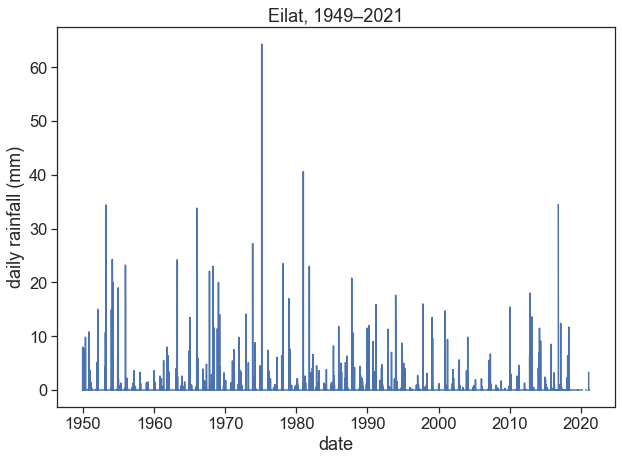Based on what you see, you might want to exclude certain periods, e.g.:

#collapse-hide

last_date = '2018-08-01'
first_date = '1950-08-01'
df = df[((df.index < last_date) & (df.index > first_date))]
df

STATION LATITUDE LONGITUDE ELEVATION NAME PRCP PRCP_ATTRIBUTES TMAX TMAX_ATTRIBUTES TMIN TMIN_ATTRIBUTES TAVG TAVG_ATTRIBUTES
DATE
1950-08-02 IS000009972 29.55 34.95 12.0 ELAT, IS 0.0 ,,E 400.0 ,,G 240.0 ,,G NaN NaN
1950-08-03 IS000009972 29.55 34.95 12.0 ELAT, IS 0.0 ,,E 410.0 ,,G 260.0 ,,G NaN NaN
1950-08-04 IS000009972 29.55 34.95 12.0 ELAT, IS 0.0 ,,E 400.0 ,,G 260.0 ,,G NaN NaN
1950-08-05 IS000009972 29.55 34.95 12.0 ELAT, IS 0.0 ,,E NaN NaN 240.0 ,,G NaN NaN
1950-08-06 IS000009972 29.55 34.95 12.0 ELAT, IS 0.0 ,,E 370.0 ,,G 240.0 ,,G NaN NaN
... ... ... ... ... ... ... ... ... ... ... ... ... ...
2018-07-27 IS000009972 29.55 34.95 12.0 ELAT, IS 0.0 ,,S 414.0 ,,S NaN NaN 359.0 H,,S
2018-07-28 IS000009972 29.55 34.95 12.0 ELAT, IS 0.0 ,,S 386.0 ,,S NaN NaN 329.0 H,,S
2018-07-29 IS000009972 29.55 34.95 12.0 ELAT, IS 0.0 ,,S NaN NaN 268.0 ,,S 334.0 H,,S
2018-07-30 IS000009972 29.55 34.95 12.0 ELAT, IS 0.0 ,,S 375.0 ,,S 277.0 ,,S 327.0 H,,S
2018-07-31 IS000009972 29.55 34.95 12.0 ELAT, IS 0.0 ,,S 390.0 ,,S NaN NaN 336.0 H,,S

24836 rows × 13 columns

The rainfall data for Eilat is VERY seasonal, it's easy to see that there is no rainfall at all during the summer. We can assume a hydrological year starting on 1 August. If you're not sure, you can plot the monthly means (see last week's lecture) and find what date makes sense best.

#collapse-hide

df_month = df['PRCP'].resample('M').sum().to_frame()
month_numbers = np.arange(1,13)
monthly_mean = np.array([])  # empty array
for m in month_numbers:      # cycle over months (1, 2, 3, etc)
this_month_mean = df_month[df_month.index.month == m].mean()  # this is the monthly mean
monthly_mean = np.append(monthly_mean, this_month_mean)    # append
# make new df and return it
df_month = pd.DataFrame({'monthly rainfall (mm)':monthly_mean,
'month number':month_numbers
})
fig, ax = plt.subplots(figsize=(10,7))
ax.bar(df_month['month number'], df_month['monthly rainfall (mm)'])
ax.set(xlabel="month",
ylabel="monthly rainfall (mm)",
title="Monthly average, Eilat, 1949--2018",
xticks=np.arange(1,13));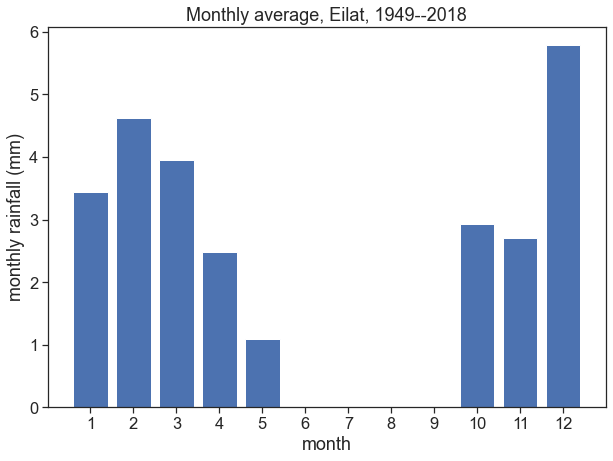Let's resample the data according to the hydrological year (1 August), and we'll keep the maximum value:

#collapse-hide

max_annual = (df['PRCP'].resample('A-JUL')
.max()
.to_frame()
)
max_annual

PRCP
DATE
1951-07-31 10.8
1952-07-31 15.0
1953-07-31 34.4
1954-07-31 24.3
1955-07-31 19.0
... ...
2014-07-31 11.5
2015-07-31 2.4
2016-07-31 8.5
2017-07-31 34.5
2018-07-31 11.7

68 rows × 1 columns

Make two graphs: a) the histogram for the annual maximum (pdf) b) the cumulative probability (cdf)

#collapse-hide

fig, (ax1, ax2) = plt.subplots(2, 1, figsize=(10,8))

h=max_annual['PRCP'].values
ax1.hist(h, bins=np.arange(0,100,10), density=True)
ax2.hist(h, bins=np.arange(0,100,10), cumulative=1, density=True)

ax1.set(ylabel="pdf")
ax2.set(xlabel="annual max (mm)",
ylabel="cdf",
);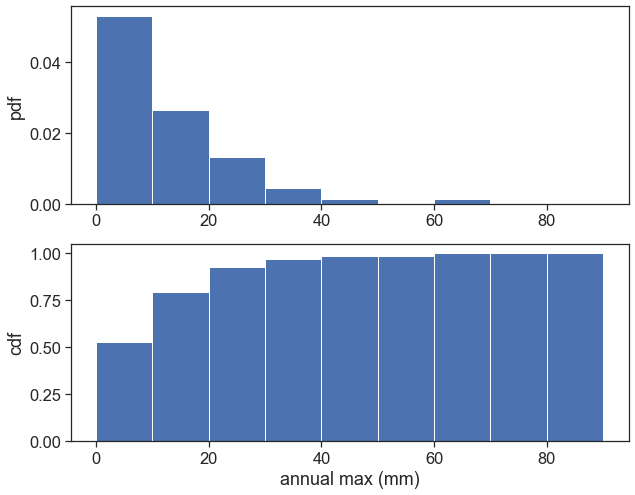Compute the plotting position and return time. You'll need to order the data in ascending order:

max_annual = max_annual.sort_values(by=['PRCP'], ascending=True)

$P_m=$ plotting position, or probability of occurence for each event
$n=$ total number of events
$m=$ rank of each event, where $m=1$ is the lowest value, and $m=n$ is the highest

Weibull plotting position:

$$P_m = \frac{m}{n+1}$$

Return period:

$$\text{Return period} = T_r = \frac{1}{1-P_m}$$

Plot the annual maximum against $P_m$ or against $T_r$.

#collapse-hide

fig, ax = plt.subplots(figsize=(10, 7))
# resample daily data into yearly data (maximum yearly value)
max_annual = df['PRCP'].resample('A-JUL').max().to_frame()
# sort yearly max from lowest to highest
max_annual = max_annual.sort_values(by=['PRCP'], ascending=True)
max_annual['rank'] = np.arange(1, len(max_annual) + 1)
print(max_annual)

n = len(max_annual['rank'])
m = max_annual['rank']
Pm = m / (n+1)
Tr = 1 / (1 - Pm)

# ax.plot(Tr, max_annual['PRCP'])
# ax.set(xlabel="return period (y)",
#        ylabel="annual maximum (mm/24h)")

ax.plot(Pm, max_annual['PRCP'])
ax.set(xlabel="non-exeedance probability",
ylabel="annual maximum (mm/24h)");

PRCP  rank
DATE
1996-07-31   0.5     1
2008-07-31   0.9     2
2000-07-31   1.2     3
2012-07-31   1.3     4
1959-07-31   1.5     5
...          ...   ...
1966-07-31  33.8    64
1953-07-31  34.4    65
2017-07-31  34.5    66
1981-07-31  40.6    67
1975-07-31  64.3    68

[68 rows x 2 columns]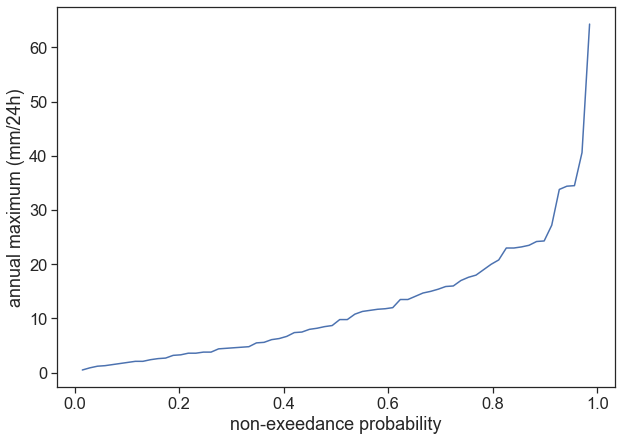Plot the annual maximum against the exceedance probability ($1-P_m$), in a log-log scale. Use

ax.set(xscale="log",
yscale("log")
)

See what data you'll want to use for a linear fit.

#collapse-hide

fig, ax = plt.subplots(figsize=(10, 6))

depth = max_annual['PRCP'].values
exc_prob = (1-Pm).values

ax.plot(exc_prob, depth, lw=3)

exclude = 40
depth_tofit = depth[exclude:]
exc_prob_tofit = exc_prob[exclude:]
ax.plot(exc_prob_tofit, depth_tofit, 'o')

ax.set(ylabel="annual maximum (mm/24h)",
xlabel="exceedance probability",
xscale="log",
yscale="log",
);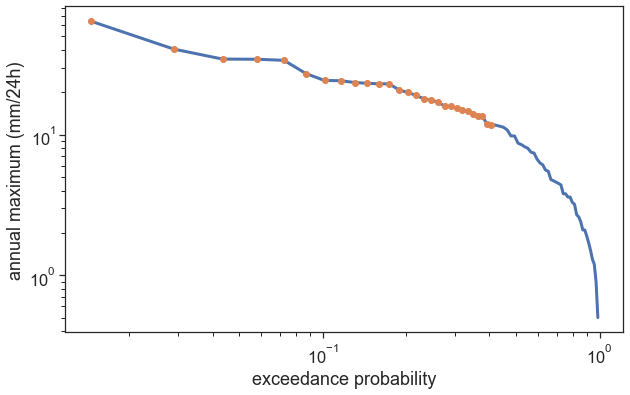Let's make a linear fit. Attention! Our data is not annual_max and exceedance_prob, but their log.

We make a linear fit using:

slope, intercept = np.polyfit(xdata, ydata, 1) # the number 1 in the order of the polynomial = linear

Write a function that receives an exceedance probability and returns the corresponding rainfall depth.

#collapse-hide

fig, ax = plt.subplots(figsize=(10, 6))

depth = max_annual['PRCP'].values
exc_prob = (1-Pm).values

ax.plot(exc_prob, depth, lw=3, label="Weibull plotting position")
ax.set(ylabel="annual maximum (mm/24h)",
xlabel="exceedance probability")
ax.set_xscale("log")
ax.set_yscale("log")

exclude = 40
depth_tofit = depth[exclude:]
exc_prob_tofit = exc_prob[exclude:]

ax.plot(exc_prob_tofit, depth_tofit, 'o')

exc_prob_tofit_log = np.log(exc_prob_tofit)
depth_tofit_log = np.log(depth_tofit)
slope, intercept = np.polyfit(exc_prob_tofit_log, depth_tofit_log, 1)

def equation(p):
return np.exp(slope*np.log(p) + intercept)
prob = [1e-3,1-1e-3]
ax.plot(prob, equation(prob), lw=3, color="tab:red", alpha=0.4)

[<matplotlib.lines.Line2D at 0x7fc1aa9feb50>]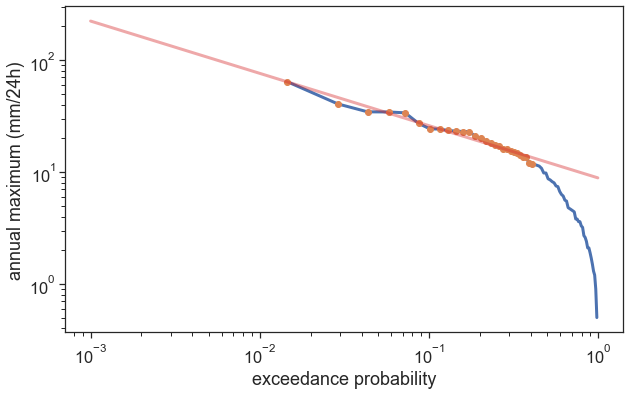Homework

Everything we did today was for 24h rainfall events. We might be interested in extreme events in longer or shorter time scales. Using the following code, calculate the return time for 3-day rainfall events:

number_of_days = 3
df2 = (df['PRCP'].rolling(number_of_days)
.sum()
.dropna()
)

All the rest after that is the same...